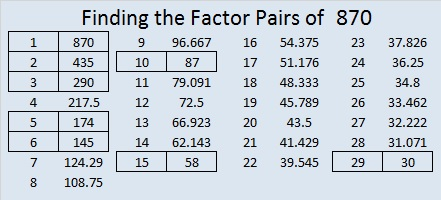# 870 If You Can Solve a 3×3 and a 4×4 Magic Square, Then You Can Solve a 12×12 Magic Square

It’s simple multiplication, folks!

3×4 = 12, so if you can solve a 3×3 Magic Square and a 4×4 Magic Square, then you can solve a 12×12 Magic Square, too.

Seriously. Really. It’s true! You really can solve a 12×12 Magic Square. I did it. Twice. You’ll see. You can do it, too.That solution consists of sixteen 3×3 Magic Squares that use all the numbers from 1 to 144. The one in the corner is the most familiar one. The other ones are just that familiar Magic Square plus 9, 18, 27 and a few other multiples of 9. I used the 4×4 Magic Square below as a guide as I placed the sixteen 3×3 Magic Squares on the excel file I made, 12 factors 864-874.make science GIFs like this at MakeaGif

There is 1 solution times 8 orientations (right-side-up, upside-down, etc.) for the 3×3 Magic Square, and there are 880 solutions times 8 orientations for the 4×4 Magic Square. That’s a lot of different possible solutions!

I also solved the 12×12 Magic Square by using nine 4×4 Magic Squares and placing them on the second grid in 12 factors 864-874. This arrangement also has many more possibilities than the one shown here.You can easily solve your own 12×12 Magic Square by opening this excel file, 12 factors 864-874, going to the 12×12 Magic Square tab, and following the directions.

Here’s more about the awesome number 870:

29 × 30 = 870. That means 870 is 29 × (29+1), so 870 is the sum of the first 29 even numbers.

870 is the sum of the ten prime numbers from 67 to 107.

Because 5 and 29 are its factors, 870 is the hypotenuse of four Pythagorean triples: ( Note that 5 × 29 = 145)

• 102-864-870, which is 6 times (17-144-145)
• 144-858-870, which is 6 times (24-143-145)
• 522-696-870, which is (3-4-5) times 174
• 600-630-870, which is (20-21-29) times 30

870 has some interesting representations in other bases:

• 606 in BASE 12, because 6(12²) + 0(12¹) + 6(12º) = 6(145) = 870
• 303 in BASE 17, because 3(17²) + 0(17¹) + 3(17º) = 3(290) = 870
• 110 in BASE 29, because 1(29²) + 1(29¹) + 0(29º) = 870
• T0 in BASE 30 (T is 29 base 10), because 29(30¹) + 0(30º) = 29(30) = 870

8 + 0 + 7 + 8³ + 0³ + 7³ = 870. Thank you OEIS.org for that fun fact.

• 870 is a composite number.
• Prime factorization: 870 = 2 × 3 × 5 × 29
• The exponents in the prime factorization are 1, 1, 1, and 1. Adding one to each and multiplying we get (1 + 1)(1 + 1)(1 + 1)(1 + 1) = 2 × 2 × 2 × 2 = 16. Therefore 870 has exactly 16 factors.
• Factors of 870: 1, 2, 3, 5, 6, 10, 15, 29, 30, 58, 87, 145, 174, 290, 435, 870
• Factor pairs: 870 = 1 × 870, 2 × 435, 3 × 290, 5 × 174, 6 × 145, 10 × 87, 15 × 58, or 29 × 30
• 870 has no square factors that allow its square root to be simplified. √870 ≈ 29.495762This site uses Akismet to reduce spam. Learn how your comment data is processed.